# Concept of Angle

• Part of Angle - Initial Side,Terminal Side,Vertex
• Types of Angle - Positive and Negative Angles
• Measuring Angles in Degrees
• initial side, terminal side, vertex,positive angle, negative angle
• Degree measure
• Relation between radian and real numbers
• Relation between degree and radian

## Definition

One complete revolution from the position of the initial side as indicated in Fig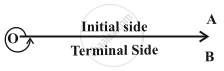## Notes

Angle is a measure of rotation of a given ray about its initial point. The original ray is called the initial side and the final position of the ray after rotation is called the terminal side of the angle. The point of rotation is called the vertex. If the direction of rotation is anticlockwise, the angle is said to be positive and if the direction of rotation is clockwise, then the angle is negative.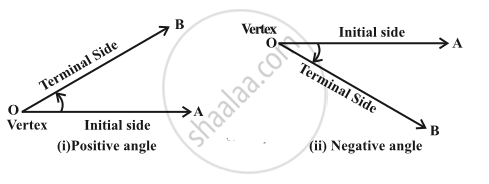Degree measure- If a rotation from the initial side to terminal side is (1/360)"th" of
a revolution, the angle is said to have a measure of one degree, written as 1°.  A degree is divided into 60 minutes, and a minute is divided into 60 seconds . One sixtieth of a degree is called a minute, written as 1′, and one sixtieth of a minute is called a second, written as 1″. Thus, 1° = 60′, 1′ = 60″ Some of the angles whose measures are 360°,180°, 270°, 420°, – 30°, – 420° are shown in Fig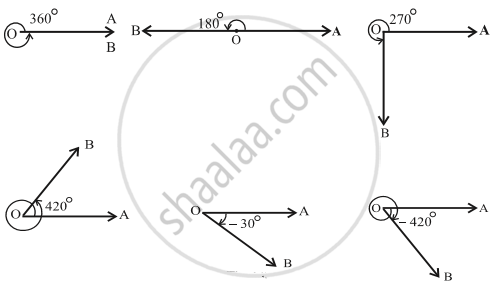Radian measure- There is another unit for measurement of an angle, called the radian measure. Angle subtended at the centre by an arc of length 1 unit in a unit circle (circle of radius 1 unit) is said to have a measure of 1 radian.
The figures show the angles whose measures are 1  radian, –1  radian, 1  1/2  radian and -1  1/2  radian.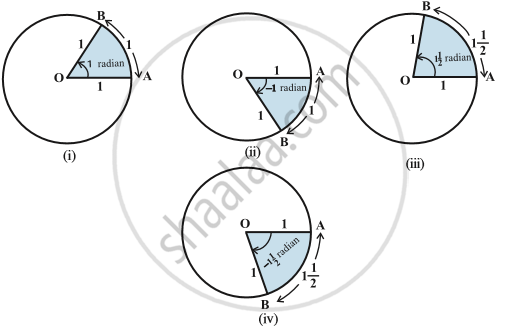Thus, if in a circle of radius r, an arc of length l subtends an angle θ radian at the centre, we have
θ= l/r or l= rθ.
Here, θ will always be represented in terms of radian.
Relation between radian and real numbers:
Consider the unit circle with centre O. Let A be any point on the circle. Consider OA as initial side of an angle. Then the length  of an arc of the circle will give the radian measure of the angle which the arc will subtend at the centre of the circle. Consider the line PAQ which is tangent to the circle at A. Let the point A represent the real number zero, AP represents positive real number and AQ represents negative real numbers (Fig). If we rope the line AP in the anticlockwise direction along the circle, and AQ in the clockwise direction, then every real number will correspond to a radian measure and conversely. Thus, radian measures and real numbers can be considered as one and the same.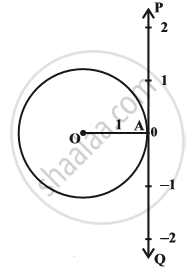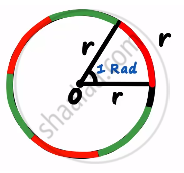Circumference of a circle =2πr
Number of arcs= 2π
π= 180°
π/2 Radians= 90°
1 Radian= "360°"/"2π" ∼ 57.2958°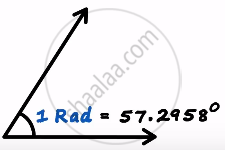The relation between degree measures and radian measure of some common angles are given in the following table: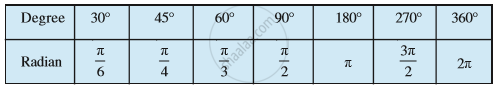Notational Convention:
Since angles are measured either in degrees or in radians, we adopt the convention that whenever we write angle θ°, we mean the angle whose degree measure is θ and whenever we write angle β, we mean the angle whose radian measure is β. Note that when an angle is expressed in radians, the word ‘radian’ is frequently
omitted. Thus, π= 180° and π/4= 45° are written with the understanding that π and π/4 are radian measures. Thus, we can say that

"Radian measure" = π/180xx "Degree measure"

"Degree measure" = 180/π xx "Radian measure"

If you would like to contribute notes or other learning material, please submit them using the button below.

### Shaalaa.com

Basics of Trigonometry 1 [00:23:47]
S
0%